# Laser Diffraction Particle Size Analyzers Need PIDS to Measure Small Particles

Laser diffraction technology is being applied in a wide range of fields. As particles become smaller, the ratio of particle dimension to light wavelength (d/λ) is reduced and the scattering pattern is smoother and less angularly dependent, causing more difficulty in determining the correct size values. To improve the ability to measure small particles, there are three approaches that can be taken to extend the lower size limit when measuring particles using laser diffraction.

The first approach to expand the lower sizing limit is by increasing the angular detecting range. If the angular location of the first minimum in the scattering pattern is used as the criterion to accurately size a sphere, to measure a sphere having a diameter smaller than 0.5 μm, the maximum detecting angle has to be greater than 90 degrees (Fig. 1). Thus, in order to size a submicron particle, the detection angular range has to be designed to cover angles at least as large as 90 degrees; practically, the maximum detecting angle can be as large as 175°.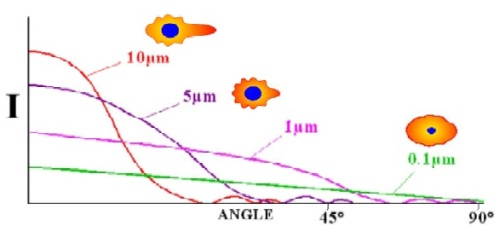Figure 1. Angle dependence on scattering pattern and the first minimum. Image credit: Beckman Coulter

Scattering patterns are function of light wavelength and particle size. Their variations are related to the ratio between particle dimension and wavelength (d/λ). Interference effects that create the fine structure in a scattering profile are greatly reduced when d/λ is lesser than 0.5. If the wavelength of light is shorter, the ratio increases and the lower sizing limit is effectively extended. In other words, scattering patterns are compressed at shorter wavelengths and more information (structure) is shown over the same angular range as compared with what can be obtained using a longer wavelength. Practically, the shortest wavelength is about 350 nm. Most materials in fact, exhibit strong absorption at wavelengths shorter than 300 nm. Using light of λ = 375 nm, the lower sizing limit can be extended to half of that obtainable by using light of λ = 750 nm.

## Polarization Intensity Differential Scattering (PIDS)

Pioneered by Beckman Coulter, most laser diffraction manufacturers use the above two approaches, i.e. wide angular detecting range and short wavelength, to size small particles. However, sizing even smaller particles which are tens of nanometers in diameter, cannot be achieved using only these two approaches. Figure 2 is a three dimensional display that shows the very slow angular variation for small particles. For particles smaller than 200 nm, even by taking benefit of the above two approaches, it is still difficult to obtain an accurate size. Then, two different routes were developed among instrument manufacturers. One is to extrapolate from the measured lower limit to an even lower limit, sometimes even beyond the theoretical lower sizing limit, e.g. 10 nm. Due to this, the data in the extrapolated region can be incorrect.

The other approach is to use the polarization effects of the scattered light. Vertically polarized scattered light has various scattering patterns and fine structures from that of horizontally polarized light for small particles. The key characteristic of the horizontal scattering intensity (Ih) for small particles is that there is a minimum of around 90 degrees. By combining polarization effects with wavelength dependence at large angles, the lower sizing limit can be extended to as low as 40 nm, almost reaching the theoretical limit. This combined approach is known as the Polarization Intensity Differential Scattering (PIDS) technique patented by Beckman Coulter.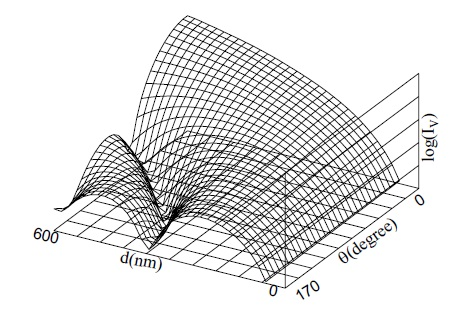Figure 2. A 3-D display of Mie scattering intensity IV from a unit volume of spheres with the relative refractive index m = 1.50 + 0i at λ = 750 nm. Image credit: Beckman Coulter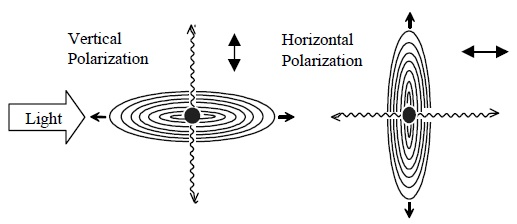Figure 3. Scattering from different polarizations. Image credit: Beckman Coulter

The origin of polarization effects can be understood in the following way (Fig. 3). When a very tiny particle, much smaller than the wavelength of light, is located in a light beam, the oscillating electric field of the light induces an oscillating dipole moment in the particle, i.e. the electrons in the atoms comprising the particle move back and forth relative to the stationary particle. The induced motion of the electrons will be in the direction of oscillation of the electric field, and therefore perpendicular to the direction of propagation of the light beam. As a result of the transverse nature of light, the oscillating dipole radiates light in all directions except in the oscillation direction; if the detector is facing the direction of oscillation it will receive no scattering from single dipoles. When the light beam is polarized in either the v direction or the h direction, the scattering intensity Iv and Ih for a given angle will be different. The difference between Iv and Ih, i.e. (Iv - Ih), is called the PIDS signal. As particle size increases, the intra-particle interference makes the particle behavior deviate from that of a simple dipole and the scattering pattern will become more complex. For tiny particles, the PIDS signal is roughly a quadratic curve centered at 90 degrees. For larger particles the pattern shifts to smaller angles and secondary peaks appear due to the scattering factor. As the PIDS signal is dependent on particle size relative to light wavelength, valuable information about a particle size distribution can be obtained by measuring the PIDS signal at several wavelengths.

Figure 4 displays the shift in the peak value and the change in contrast for particles of various diameters. Furthermore, because the PIDS signal varies at different wavelengths, measurement of the PIDS signals at several wavelengths will offer additional scattering information that can be used to further refine the size retrieval process.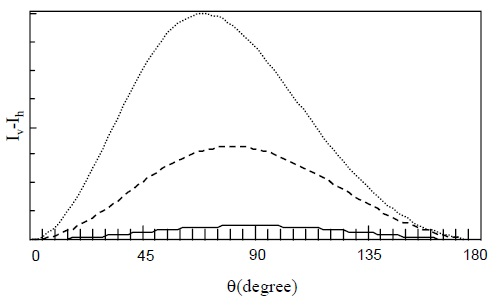Figure 4. Iv-Ih of small PSL in water (λo = 450 nm). Dotted line: d = 150 nm; dashed line: d = 100 nm; and solid line: d = 50 nm. Image credit: Beckman Coulter

From Figure 4, the angular patterns for 100 nm and even for 50 nm particles are recognizable, in addition to the shift in the axis of symmetry. It has been verified through both theoretical simulation and real experimentation that accurate sizing of particles smaller than approximately 200 nm by scattering intensity without the use of the PIDS technique is difficult and probably unrealistic. The combination of the three approaches (wider angular range, wavelength variation, and polarization effects) enhances the accurate characterization of submicron particles using light scattering.

Figure 5 is a typical trimodal distribution retrieved in a laser diffraction experiment using the PIDS technique at multiple wavelengths (λo = 450, 600, 750, and 900 nm) and over a scattering angular range with angles up to 144 degrees, with (solid line) and without (dashed line) using the polarization effect. The dotted lines show the nominal diameter values of the latexes in the mixture as reported by the PSL vendor. Without the PIDS technique the smallest component is missed, even when using the information gathered at large scattering angles and short wavelengths. Figure 6 is a scanning electron microscopy (SEM) image of the sample analyzed in Figure 5 in which three different sizes of particles can be seen.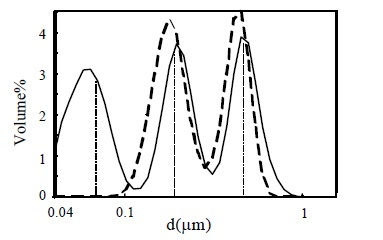Figure 5. A trimodal mixture of PSL (nominal diameters 83 nm, 204 nm, and 503 nm with 1:1:1 volume ratio). Image credit: Beckman Coulter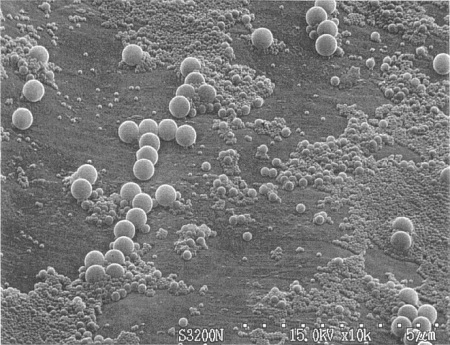Figure 6. An electron microscopic image of the sample in Figure 5. Image credit: Beckman Coulter

## Conclusions

By using all three approaches, i.e. wide angular range, short wavelength, and polarization effect, particle size as small as 40 nm can be correctly measured instead of being "extrapolated". There is no mixing of technologies. All signals are from the same scattering phenomenon and treated integrally in a single data retrieval process just like in an ordinary laser diffraction measurement.This information has been sourced, reviewed and adapted from materials provided by Beckman Coulter, Inc. - Particle Characterization.